From last few days, I was writing posts on Fun Maths Brain Teasers. Now let me post some brain teasers which will require logical thinking. These are the picture puzzles in which an object is equated to a number. One has to come up with the logical deduction of this equation and then applying the same rules have to solve for the last object.
These logical picture brain teasers first require logical thinking for relating the object to numbers and then mathematical calculations to compute the value of the object. This brain teaser requires knowledge of the English language too.  I have created these logical brain teasers for feedback. If one loves these puzzles, please do write your comments with your feedback in the comments section of this post. Depending upon the feedback regarding these puzzles, I will be creating some more puzzles of these types. Different people may interpret these brain teasers differently. In case your logic is different from what is mentioned here, please do post your answer with explanation. We will love to read the different views :)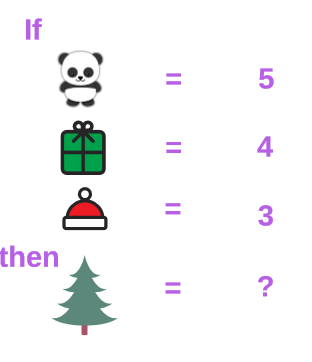1. Logical Pictures Brain Teasers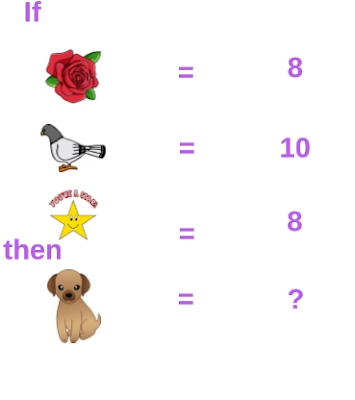2. Logical Pictures Brain Teasers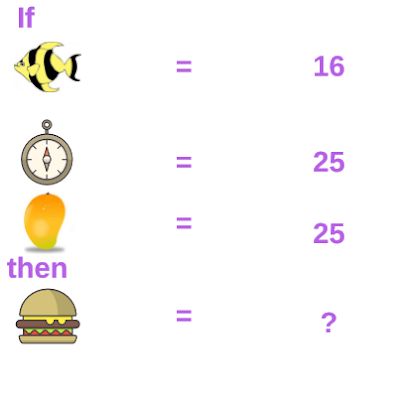3. Logical Pictures Brain Teasers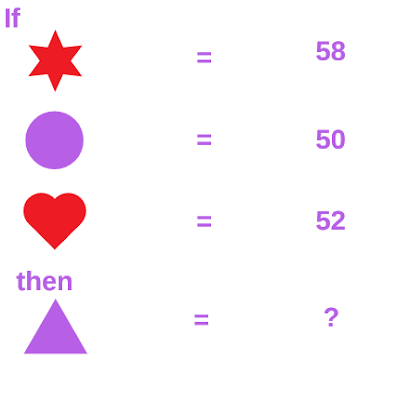4. Logical Pictures Brain Teasers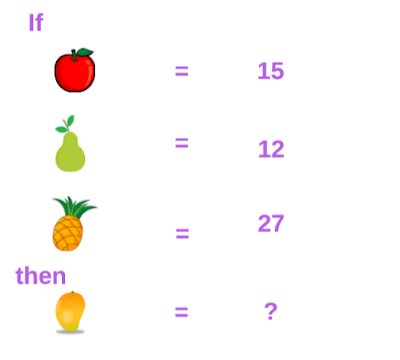5. Logical Pictures Brain Teasers

These Fun Riddles are part of Logical Brain Teasers. Below are some similar puzzles which you should check to challenge your brain.
List of Logical Brain Cracking Puzzles

1) What Comes Next In The Sequence Riddles With Answers: It contains the logical and mathematical sequence puzzles in which your challenge is to find the next number in the series.

2) Will you crack the code? puzzles with answers: These are purely logical puzzles in which you challenge to find the 3 digit code and then open the lock.

3) River Crossing Challenges with answers: In these puzzles, you have to use your logical reasoning to help a few people who want to cross the river.

4) Very Tricky Brain Cracking Questions for adults with answers: These are very tricky and tough logical questions which will crack your brain. If you consider yourself genius then do try out these puzzles.

Hidden Answers to these Logical Pictures Brain Teasers is given below. Do select the text between the numbers to look at the given answer.
------------------
A1.
Panda has five alphabets,
Cap has three alphabets
The tree has four alphabets

A2.
Rose  = 4 x 2= 8
Star = 4x2 =8
Pigeon = 5x2 =10
Dog = 3x2= 6

A3.
Fish = 4x4 = 16
Clock = 5x5 = 25
Mango = 5x5= 25
burger = 6x6 = 36

A4.
Sum of the alphabetical position of the word
First is a star, circle, heart, triangle.

A5.
Apple = 5x3= 15
Pear = 4x3 =12
Pineapple= 9x3 =27
Mango = 5x3=15